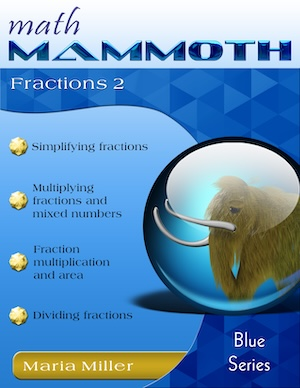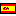^
You are here: HomeVideos → Fraction videos, part 2

# Free fraction videos online

Find here free online math videos on these fraction topics:

(Fraction videos, part 1, are on this page.) The videos are recorded in high-density (HD) and are viewable both here as well as at my Youtube channel.

These videos are usable for students, teachers, and parents. You can use them...

• To learn these topics yourself (if you're a student for example, or an adult needing a refresher)
• As lesson plans for teaching these topics. Often, one video from below can be made into several lessons with students.

The videos match the lessons in my book Math Mammoth Fractions 2 (Blue Series book), or the lessons in chapter 6 of Grade 5-B (Light Blue series). In either book, you will get MANY more practice exercises, word problems, and puzzles than what are shown in the videos, and also some lessons that are not in covered in this set of videos.119 pages (includes answers) PDF download \$5.75 Printed copy \$10.20 Math Mammoth Fractions 2 worktext A self-teaching worktext that focuses on simplication, multiplication, and division of fractions & mixed numbers, among other topics. => Learn more!

## Simplifying fractionsFirst I show the simplification process using visual models and an arrow notation to help students understand the concept. Simplifying fractions is like joining or merging fractional pieces together, such as in 4/12, we merge each 4 pieces so we get 1/3.

I show how we can simplify a fraction in several steps, instead in one step. If you simplify in one step, you need to use the greatest common factor of the numerator and denominator, but this is not necessary if you simplify in several steps.

Sometimes you cannot simplify. Lastly we explore if the given fractions are already in their lowest terms.

## Multiply fractions by whole numbersMultiplying fractions by whole numbers is a fairly easy concept. Students just need to remember that 4 × (2/3) is not calculated as (4 × 2) / (4 × 3). In the visual model, you can color two thirds, four times, to get the answer. Doubling or tripling recipes is a nice application of this concept.

I also show an interesting connection between (1/3) × 5 or one-third of five pies, and 5 × (1/3), or five copies of 1/3.

Practice this online: multiply fractions by whole numbers

## Multiply fractions by fractionsI start out by explaining that (1/2) × (1/3) means 1/2 of 1/3, and we find that visually. Similarly, (1/3) × (1/4) means 1/3 of 1/4. From this we get a shortcut that (1/m) × (1/n) = (1/mn).

Next, we find what is 2/3 of 1/4. First, we find 1/3 of 1/4 as being 1/12. Therefore, 2/3 has to be double that much, or 2/12.

After introducing the shortcut for fraction multiplication (multiply the numerators, multiply the denominators), I solve a few simple multiplication problems and a word problem.

Lastly, I justify the common rule for fraction division (sort of a "proof" for fifth grade level).

Practice this online: multiply fractions by fractions

## Simplifying before multiplying - fraction multiplicationI explain how we can simplify before we multiply fractions, and also why we are allowed to do so.

## Multiplying mixed numbersMultiplying mixed numbers is easy: simply convert them to fractions first, then multiply using the shortcut (rule) for fraction multiplication.

The difficulty comes in remembering to change them to fractions!

Some students have misconception that you can multiply the whole-number parts and the fractional parts separately, such as (1 1/2) × (1 1/2) = 1 1/4. I show with an area model why that is wrong.

Lastly, I solve a word problem involving area of a rectangle and a square.

Practice this online: multiply mixed numbers by mixed numbers

## Fraction multiplication and areaI explain how fraction multiplication and area of rectangles relate to each other. Basically, if the sides of the rectangle are fractional parts of a unit, then we solve the area by multiplying the fractions (of course). And, the visual model provides a neat illustration of fraction multiplication.

## Divide fractions: mental mathI show two situations where we can divide fractions without using the usual "rule" for fraction division, but just mental math.

The first is when we divide a fraction by a whole number and we can think of pie pieces shared evenly between so many people, such as 6/10 divided by 3.

The other situation is a fraction divided by a fraction, and has to do with thinking "How many times does the divisor 'fit' into the dividend?" such as for example 4/7 divided by 2/7 (two times).

## How to divide fractions & reciprocal numbersI explain what reciprocal numbers are, including a visual interpretation for them. Then we study the rule for fraction division: To divide by a fraction, multiply by its reciprocal.

Lastly, I explain why this rule works, based on reciprocal numbers and on interpreting division as "how many times does the divisor fit into the divi

## Fractional part of a groupThis is sort of a "lesson plan" for finding a fractional part of a group of objects, or a fractional part of a number. Basically, 1/3 of 18 is a division problem 18 divided by 3. (To find a fractional part of a number when the fraction is of the form 1/n, just divide by n.) And to find 5/11 of 44, first find 1/11 of 44, which is 44 divided by 11 = 4. Then, multiply that times 5.

## Ratios and fractionsA ratio is a comparison of two numbers (or quantities) using division. For example, if I have 4 hearts and 3 stars, then the ratio of hearts to stars is 4:3 (four to three).

We can often "translate" between ratio language and fraction language.

Lastly I work several word problems that involve ratios and fractions, using a bar (or block) model, a powerful visual aid that helps students from grade 4 onward solve problems that would otherwise require algebra.

## How to convert fractions into decimalsSome fractions we can convert first to equivalent fractions with a denominator 10, 100, 1000 etc. and then from those, into decimals. But most often, to convert a fraction into a decimal, we need to divide (long division or calculator).

For example, to convert 5/7 into a decimal, divide 5 by 7.

Sometimes, in such a division, the decimal ends. More often though, it is a nonending repeating decimal. We see that when in our long division the same remainders keep coming up in the same order.

Such decimals repeat part of their decimal digits, such as 0.13131313.... or 0.83567567567567... (567 repeats)

You might ask if decimals that don't repeat exist. Yes, they do. They are irrational numbers, meaning they are NOT fractions (not rational numbers), and they are quite a fascinating topic in themselves.

## Divide fractions: an alternative algorithmIn this alternative algorithm for fraction division we first convert the two fractions to have the same denominator (like fractions). Then, we simply divide the numerators, so it becomes a whole-number division. I also show a proof of this method.

See also free fraction videos, part 1: mixed numbers, equivalent fractions, adding and subtracting fractions & mixed numbers.

The videos match the lessons in my book Math Mammoth Fractions 2 (Blue Series book), or the lessons in chapter 7 of Grade 5-B (Light Blue series). In either book, you will get MANY more practice exercises, word problems, and puzzles than what are shown in the videos, and also some lessons that are not in covered in this set of videos.119 pages (includes answers) PDF download \$5.75 Printed copy \$10.20 Math Mammoth Fractions 2 worktext A self-teaching worktext that focuses on simplication, multiplication, and division of fractions & mixed numbers, among other topics. => Learn more!

By Maria Miller

WAIT!

Receive my monthly collection of math tips & resources directly in your inbox — and get a FREE Math Mammoth book!We respect your email privacy.
You can unsubscribe at any time.

### Math Mammoth Tour

Confused about the different options? Take a virtual email tour around Math Mammoth! You'll receive:

An initial email to download your GIFT of over 400 free worksheets and sample pages from my books. Six other "TOURSTOP" emails that explain the important things and commonly asked questions concerning Math Mammoth curriculum. (Find out the differences between all these different-colored series!)

This way, you'll have time to digest the information over one or two weeks, plus an opportunity to ask me personally about the curriculum.
A monthly collection of math teaching tips & Math Mammoth updates (unsubscribe any time)We respect your email privacy.

Note: You will FIRST get an email that asks you to confirm your email address. If you cannot find this confirmation email, please check your SPAM/JUNK folder.

### "Mini" Math Teaching Course

This is a little "virtual" 2-week course, where you will receive emails on important topics on teaching math, including:

- How to help a student who is behind
- Troubles with word problems
- Teaching multiplication tables
- Why fractions are so difficult
- The value of mistakes
- Should you use timed tests
- And more!

You will also receive:

A GIFT of over 400 free worksheets and sample pages from my books right in the very beginning.A monthly collection of math teaching tips & Math Mammoth updates (unsubscribe any time)
We respect your email privacy.

Note: You will FIRST get an email that asks you to confirm your email address. If you cannot find this confirmation email, please check your SPAM/JUNK folder.

### Maria's Math Tips

Enter your email to receive math teaching tips, resources, Math Mammoth news & sales, humor, and more! I tend to send out these tips about once monthly, near the beginning of the month, but occasionally you may hear from me twice per month (and sometimes less often).You will also receive:

• A GIFT of over 400 free worksheets and sample pages from my books.We respect your email privacy.